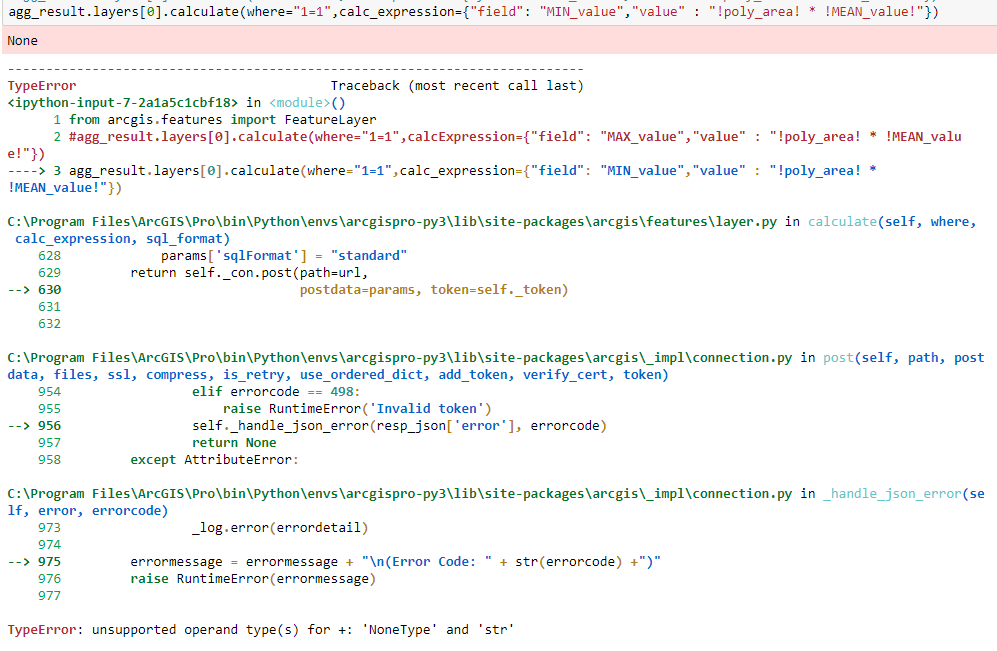# arcgis.features.FeatureLayer --> calculate

369
0
07-19-2017 08:39 AMbyEsri Contributor

Hi,

I would like to calculate a field value using the

`   calculate`(where, calc_expression, sql_format=’standard’)

operation of the `arcgis.features.``FeatureLayer `class.

I have to fields that I want to multiply but

agg_result.layers.calculate(where="1=1",calc_expression={"field": "MIN_value","value" : "!poly_area! *    !MEAN_value!"},sql_format="standard")

is throwing the following error: TypeError: unsupported operand type(s) for +: 'NoneType' and 'str'The CalculateField operation of arcpy is workinf fine:

arcpy.management.CalculateField(agg_result.layers.url, "MAX_value", "!poly_area! * !MEAN_value!",    "PYTHON_9.3", None)

Can anyone tell me what I'm doing wrong?

Thanks!

Thomas

Tags (1)
0 Replies